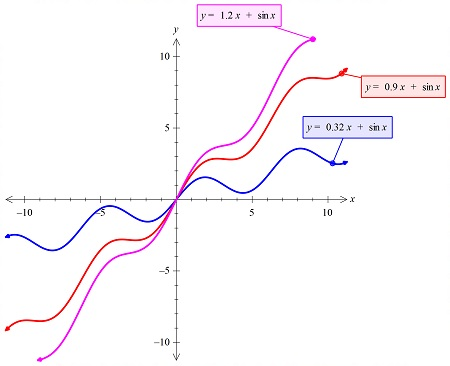# Graph the f(x)=kx+sin(x) \ for \ k=.32 , 0.9 \ and \ 1.2 What are the differences between the...

## Question:

Graph the {eq}f(x)=kx+sin(x) \ for \ k=.32 , 0.9 \ and \ 1.2 {/eq}

What are the differences between the graphs?

## Graphs:

The graph of the function that has the arbitrary constant term in it, will vary as the arbitrary constant value is changed. The graph may either bend toward the y-axis of may bend away from the y-axis.

## Answer and Explanation:

The three graphs are plotted in the same figure below:So we see that as the value of k is increasing the graph is bending more toward the y-axis.

#### Learn more about this topic:Graphs: Types, Examples & Functions

from High School Algebra II: Help and Review

Chapter 16 / Lesson 11
355K